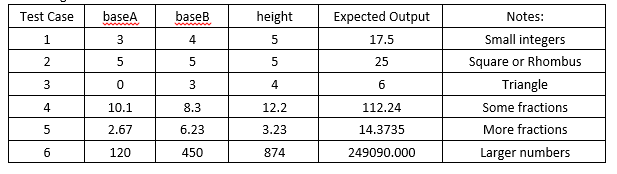# [Solved] CMIS102-Lab3-Trapezoid araa Overview

Click And Check all our Assignments

10 USD \$

Category: Tag:

Week 3

This hands-on lab allows you to follow and experiment with the critical steps of developing a program including the program description, analysis, test plan, design, pseudocode visualization, and implementation with C code. The example provided uses mathematical operators and variable types.

Program Description

This program will calculate the area of a trapezoid. The program will ask the user to enter the two bases and height and then use these values to calculate and then print the area of the trapezoid. The design step will include pseudocode.

Analysis

I will use sequential programming statements.

I will define three float numbers for the two bases and the height: baseA, baseB, height. Float numbers were selected as opposed to integers to make sure trapezoids of all dimensions are possible and not just whole numbers. Float number will store the area: area

The area will be calculated by this formula:

Area = ½ * (baseA + baseB) * height

For example if the bases are 4.7 and 2.1, and the height is 5.3 the area is calculated as:

Area = ½ * (4.7 + 2.1) * 5.3 = ½ * 6.8 * 5.3 = 3.4 * 5.3 = 18.02

Test Plan

To verify this program is working properly the following baseA, baseB and height values could be used for testing:Pseudocode

// This program will calculate the area of a trapezoid.

// Declare variables

Declare baseA, baseB, height, area as Float

Write “Enter the first trapezoid base:”

Input baseA

Write “Enter the second trapezoid base:”

Input baseB

Write “Enter triangle height:”

Input height

// Set value of area

Set area=1/2*(baseA + baseB) * height

// Print area

Print “Area of the trapezoid is “ + area

C Code

The following is the C Code that will compile in execute in the online compilers.

// C code

// This program will calculate the area of a trapezoid.

// Developer: Faculty CMIS102

// Date: Jan 31, XXXX #include <stdio.h>

int main ()

{

/* variable definition: */ float baseA, baseB, height, area;

/* Prompt user for baseA */

printf(“Enter the first base of the trapezoid:
”);

// Input the base scanf(“%f”, &baseA);

/* Prompt user for baseB */

printf(“Enter the second base of the trapezoid:
”);

// Input the baseB scanf(“%f”, &baseB); /* Prompt user for height */

printf(“Enter the height of the trapezoid:
”);

// Input the height scanf(“%f”, &height); // Calculate the Area

area= 0.5 * (baseA+ baseB) * height;

// Print the result printf(“Area is : %f
”, area); return 0;

}

Setting up the code in repl.it:

And the conversation panel:

Note the bases and height (4.7, 2.1 and 5.3) are entered in as responses to the prompts. You can change these values to any valid float values to match your test cases.

Learning Exercises for you to complete

1. Demonstrate you successfully followed the steps in this lab by preparing screen captures of you running the lab as specified in the Instructions above.
2. Change the C code to calculate the perimeter of the corresponding right trapezoid, where two of the angles are 90°. Support your experimentation with a screen capture of an execution of the new code. A little geometry, using the Pythagorean Theorem, will derive the following formula for the perimeter:

P = baseA + baseB + height + squareRoot (height^2 + (baseA – baseB)^2) Which can be coded as:

perimeter = baseA + baseB + height

sqrt (height*height + (baseA – baseB)*(baseA – baseB))

NOTE: The code will also need the following include statement added just after the

“#include <stdio.h>“ line in the code:

#include <math.h>

1. Prepare a new test table with at least 3 distinct test cases listing input and expected output for the perimeter of a right trapezoid.
2. What is this line of code doing?

scanf(“%f”, &height);

How would you change this line if you wanted to input an Integer as opposed to a float?

1. What are the values of g and h after executing the following C code?

#include <stdio.h>

int main(void) { int i,j; float f,g,h;

i = 5; j = 2; f = 3.0; g = f + j / i; h = (f + j)/i;

printf(“values of g,h are %f,%f
”, g,h); return 0;

}

Describe specifically, and in your own words, why are the values of g and h different? Support your experimentation with a screen capture of an execution of the code.

## Reviews

There are no reviews yet.

Only logged in customers who have purchased this product may leave a review.

Shopping Cart[Solved] CMIS102-Lab3-Trapezoid araa Overview
10 USD \$